# Exponential, Logarithmic, Sine, and Cosine Integrals

by on November 22, 2011

The Multiprecision Computing Toolbox version 3.1.6 introduces multiple new routines for computing special functions in arbitrary precision:

expint(z, n) % Exponential Integral, En(z) eint(x) % Exponential Integral (in terms of Cauchy principal value) logint(x) % Logarithmic Integral cosint(z) % Cosine Integral sinint(z) % Sine Integral chint(z) % Hyperbolic Cosine Integral shint(z) % Hyperbolic Sine Integral

The specific details of the implementation are given below.

## Exponential Integrals

expint(z [,n]) computes the generalized exponential integral defined by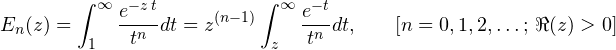If no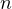is supplied, the routine computes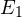by default (to match MATLAB’s built-in expint):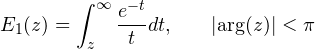eint(x) gives the exponential integral in terms of the Cauchy principal value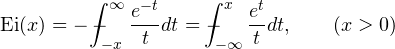## Logarithmic Integral

The function logint(x) calculates the logarithmic integral related to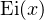as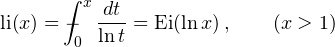## Sine and Cosine Integrals

cosint(z), sinint(z) compute the trigonometric integrals defined by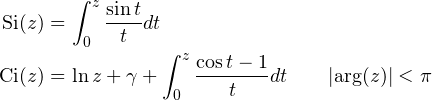chint(z), shint(z) provide hyperbolic analogs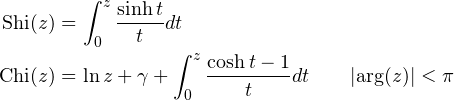## Implementation

All of the new functions are implemented using convergent series for small arguments. Continued fractions are used to evaluate the functions for larger arguments (by Lentz’s algorithm). As a result, computations are fast and stable, even for extremely large operands:

>> mp.Digits(50); >> format short g   >> expint(mp('(1-i)*10^6')) 2.1210911712154725095282810380420777177470925995558e-434301 + 9.672206089116328709021760942950103877176245369514e-434302i   >> tic; expint(mp('(1-i)*10^6')); toc; Elapsed time is 0.002218 seconds.

The implementation of the hyperbolic integrals chint(z), shint(z) is only preliminary, they have not been finalized yet. Please note that at this time they can be unstable.